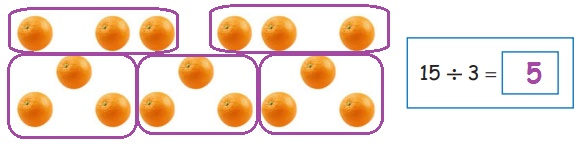Home | | Maths 3rd Std | Exercise (Equal Grouping)

# Exercise (Equal Grouping)

Text Book Back Exercises Questions with Answers, Solution

Exercise

a. Divide 8 balls into a group of 2 each.8 ÷ 2 = 4

b. Divide 15 oranges into a group of 3 each.15 ÷ 3 = 5

c. Divide 20 cups into groups of 5 each.20 ÷ 5 = 4

Activity

* Give a handful of tamarind seeds to a child and keep some number chits in a box. Let him/her pick out a number chit from the box.

* Now let him/her make it to groups as per the number in the chit taken from the box.

* Once the child has finished grouping ask him/her to write the division fact on the blackboard.

Tags : Numbers | Term 3 Chapter 2 | 3rd Maths , 3rd Maths : Term 3 Unit 2 : Numbers
Study Material, Lecturing Notes, Assignment, Reference, Wiki description explanation, brief detail
3rd Maths : Term 3 Unit 2 : Numbers : Exercise (Equal Grouping) | Numbers | Term 3 Chapter 2 | 3rd Maths